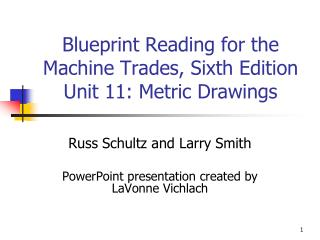DownloadDownload PresentationBlueprint Reading for the Machine Trades, Sixth Edition Unit 11: Metric Drawings

# Blueprint Reading for the Machine Trades, Sixth Edition Unit 11: Metric Drawings

Télécharger la présentation## Blueprint Reading for the Machine Trades, Sixth Edition Unit 11: Metric Drawings

- - - - - - - - - - - - - - - - - - - - - - - - - - - E N D - - - - - - - - - - - - - - - - - - - - - - - - - - -
##### Presentation Transcript

1. Blueprint Reading for the Machine Trades, Sixth Edition Unit 11: Metric Drawings Russ Schultz and Larry Smith PowerPoint presentation created by LaVonne Vichlach

2. Metric Drawings • More common today • Shown on the print in a variety of ways • Effort made to use whole or half millimeters except for standard tools • Metric conversion-multiply by .03937 for inches • Best to think metric

3. Metric Drawings • First to have symbols precede dimension figures • Both metric and inch systems follow this organization today

4. Dimensioning Symbols • Major move toward international standardization of symbols • Symbols have meanings

5. Metric Threads • System similar to Unified threads • Major diameter is first after prefix “M” • Pitch tolerance class specification • Only two series of thread are standard in metric system • Coarse • Fine

6. Metric Threads • Tolerance Class • Numbers and letters designate tolerance for pitch • Major diameters for external • Minor diameters for internal

7. Metric Threads • Tolerance Class • Letters indicate the position of thread tolerance in relation to basic diameter • “e” is large allowance • “g” is a small allowance • “h” is no tolerance

8. Metric Threads • Tolerance Class • 6H/6g are general purpose • Comparable to U.S. 2A/2B classes of fit • May be assumed when omitted from specifications • 4H5H/4h6h similar to U.S. class 3A/3B

9. Flanged Hub 1. Are the units of measurement in (a) inches, (b) millimeters, or (c) centimeters? (b) millimeters

10. Flanged Hub 2. Is the section view a (a) half-section or a (b) full section? (b) full

11. Flanged Hub 3. What tolerance applies to the flange diameter? ±1

12. Flanged Hub 4. What is the MMC of the bore diameter? 25.4

13. Flanged Hub 5. Write the flange diameter in limit form. 99 -101

14. Flanged Hub 6. Write the bore diameter with bilateral tolerance. 25.45 ±0.05

15. Flanged Hub 7. Convert the flange diameter to its decimal inch equivalent. 3.397

16. Flanged Hub 8. What is the diameter of the bolt circle? 82.6

17. Flanged Hub 9. What is the diameter of the spotface? 19

18. Flanged Hub 10. Does the spotface run tangent with the diameter of the flange? No

19. Flanged Hub 11. What is the major diameter of the threads? 10

20. Flanged Hub 12. What is the pitch of the threads? 1.5

21. Flanged Hub 13. What is the tolerance class of the threads? 6H

22. Flanged Hub 14. Are the threads coarse or fine? Coarse

23. Flanged Hub 15. How many full threads will the tapped hole contain? 11

24. Flanged Hub Calculate the dimension for the letter A 45°

25. Flanged Hub Calculate the dimension for the letter B 41

26. Flanged Hub Calculate the dimension for the letter C 20

27. Flanged Hub Calculate the dimension for the letter D 8

28. Flanged Hub Calculate the dimension for the letter E 4.25

29. Flanged Hub Calculate the dimension for the letter F 8.7

30. Flanged Hub Calculate the dimension for the letter G 37

31. Flanged Hub Calculate the dimension for the letter H 6.8

32. Flanged Hub Calculate the dimension for the letter I MIN 16.75

33. Flanged Hub Calculate the dimension for the letter J MAX 15.9

34. Metric Hole Block 1. What units of measurement are used for dimensioning? Millimeters

35. Metric Hole Block 2. What do the parentheses enclosing some of the dimensions designate? Reference

36. Metric Hole Block 3. Are the holes located by chain dimensioning or datum dimensioning? Datum

37. Metric Hole Block 4. What is the maximum tolerance accumulation between any two hole locations? ± 0.4

38. Metric Hole Block 5. Is the specified material an alloy steel or a carbon steel? Carbon Steel

39. Metric Hole Block 6. Is the specified material a high-, medium, or low –carbon steel? Low-Carbon

40. Metric Hole Block 7. What tolerance class is specified for the threaded holes? 6H

41. Metric Hole Block 8. What is the major diameter of the smallest threaded hole? 6

42. Metric Hole Block 9. Are the M8 threads coarse or fine? Coarse

43. Metric Hole Block 10. What is the thread pitch of the blind hole? 1.5

44. Metric Hole Block 11. What is the tolerance on the depth of the tap drill in the blind hole? ±1.5

45. Metric Hole Block 12. What is the chamfer angle on the threaded holes? 45°

46. Metric Hole Block 13. Calculate the minimum number of full threads possible in the blind hole. (disregard chamfer) 10

47. Metric Hole Block 14. How far apart are the countersunk hole and the counterbored hole? 22

48. Metric Hole Block 15. How far apart are the spotfaced hole and the M6 threaded hole? 27

49. Metric Hole Block 16. What is the diameter of the spotface? 22